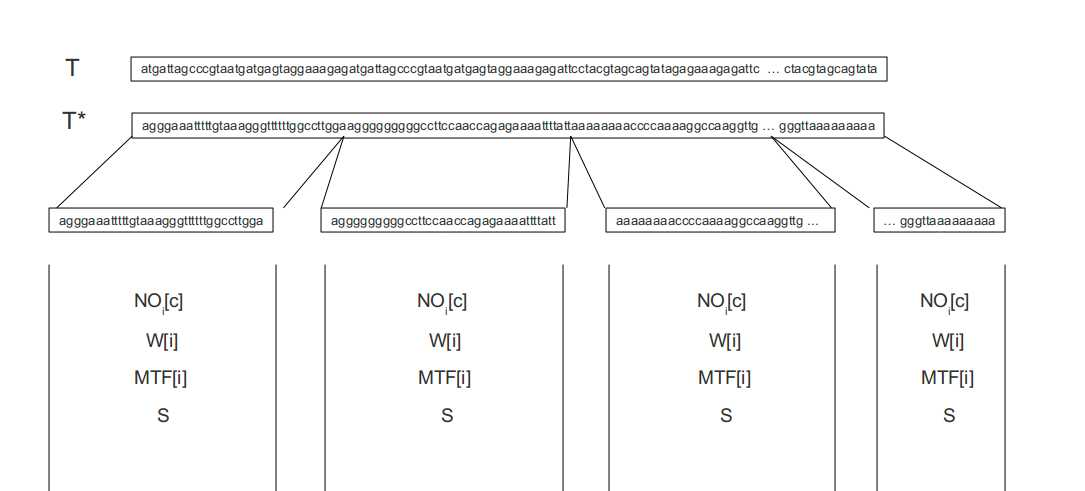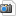You are here: Foswiki>ABI Web>ThesesHome>FMIndex (27 Jun 2011, JochenSinger)

# Page FMIndex

## TITLE

`TITLE`

Student
Jochen Singer

Prof. Dr. Knut Reinert, David Weese

## Expose

Recent developments in the field of DNA research have reduced the cost of genome sequencing drastically. While the analyses of individual genomes was very expensive in the past, leading to only a few studies, the numbers of studies has increased by magnitudes recently. In addition to the increase in studies, the amount of data per study has increased dramatically as well. Therefore fast and at the same time space efficient analysis algorithms have to be developed.

One such approach is the FM-Index. The index is based on the Burrows-Wheeler transformation (BWT) which is a rearrangement of a given text T into T* (BWT(T) = T*). The transformation is computes using a suffix array. In doing so the BWT rearranges T in such a way that T is usally more compressible than T. Furthermore one can efficiently search for patterns P in T or count them. In order to do so some auxiliary data is needed:
• occ - occ('c',loc) = how many occurrences of c are found in T* up to position loc
• c('c') = total number of characters lexicographically smaller than c

This project focuses on the implementation of the FM-Index. More precisely we will implement:
• The Burrows-Wheeler transformation (this should already be included in SeqAn)
• The auxiliary tables occ, c and sparseSA (I still need to find out what precisely the sparseSA is): A first version will be a naive implementation which does not include the compression of occ. Afterwards a more sophisticated implementation focusing on the reduction of space consumption will be implemented. In order to do so different approaches will be compared and one chosen which shows a reasonable trade off between time and space consumption.

Compression (this paragraph has been copied from , page 558)
• Use move-to-front encoding to replace each character of T* with the number of distinct characters seen since its previous occurrence. the encoder maintains a list, called the MTF-list, initialized with all characters of ordered alphabetically. When the next character arrives, the encoder outputs its current rank in the MTF-list and moves it to the front of the list. At any instant, the MTF-list contains the characters ordered by recency of occurrence. Note that runs of identical characters in L are transformed into runs of zeros in the resulting string Lmtf = mtf(T*). The string Lmtf is defined over the alphabet {0, 1, 2, . . . , |Sigma| - 1}.
• Encode each run of zeros in L mtf using a run-length encoder. More precisely, replace any sequence 0m with the number (m + 1) written in binary, least significant bit first, discarding the most significant bit. For this encoding, we use two new symbols 0 and 1. For example, 05 is encoded with 01, and 07 is encoded with 000. Note that the resulting string L rle = rle(Lmtf ) is defined over the alphabet { 0, 1, 1, 2, . . . , |Sigma| -1}.
• Compress Lrle with the following variable-length prefix code. Encode 0 and 1 using two bits (10 for 0, 11 for 1). For i = 1, 2, . . . , |Sigma| - 1, encode the symbol i using 1 + 2 log(i + 1) bits: log(i + 1) 0's followed by the binary representation of i + 1 that takes 1 + log(i + 1) bits. The resulting string is the final output of algorithm BW_RLX and is defined over the alphabet {0, 1}.

Searching

The bottleneck in terms of speed is the occ function. The function is used to search in the compressed text and can be computed efficiently by creating buckets which cover the whole sequence and store some auxiliary data. The figure below is a graphical representation of the described situation.• First of all the original text T is converted into T* (T*=bwt(T)).
• T* is divide into several buckets each of size l.
• A table NO for each bucket is created which stores how many occurrences of 'c' have been counted before the start of the current bucket. For example: NO3[c]= 222 would mean that there are 222 occurrences of c before the start of the current bucket.
• W[i] states the size of the buckets up to the current bucket
• MTF[i] = Move to Front, stores a picture of the MTF-list (see compression) at the beginning of the current bucket
• S[c, h, BZi, MTF[j]] stores the number of occurrences of c among the first h charactes of the compressed bwt of the current bucket. BZi is the compressed bwt of the current bucket i and MTF[j] is a picture of the MTF-list at the start of the current bucket.

In addition to the structure described above the tables NO and W are stored in superbuckets of size lb.

In order to determine occ(x,'c') one has to determine which bucket contains the position x. Afterwards one adds the entries from the superbuckets and buckets before the one which contains x. The current bucket is then decompressed and the cs before x are counted. Using sophisticated data structures, such as wavelet trees, this can be done in constant time.

The implementation will be done in such a way that the Finder interface of SeqAn can be used. In addition the implementation should support the use of a string set.

## Literature

 M. Burrows and D. J. Wheeler (1994) A Block-sorting Lossless Data Compression Algorithm, SRC Research Report 124
 G. Navarro and V. Ma ̈kinen (2007) Compressed Full-Text Indexes, ACM Computing Surveys 39(1), Article no. 2 (61 pages), Section 5.3
 D. Adjeroh, T. Bell, and A. Mukherjee (2008) The Burrows-Wheeler Transform: Data Compression, Suffix Arrays and Pattern Matching, Springer
 P. Ferragina, G. Manzini (2000) Opportunistic data structures with applications, Proceedings of the 41st IEEE Symposium on Foundations of Computer Science
 P. Ferragina, G. Manzini (2001) An experimental study of an opportunistic index, Proceedings of the 12th ACM-SIAM Symposium on Discrete Algorithms, pp. 269-278
 P. Ferragina, G. Manzini (2005) Indexing compressed text, Journal of the ACM, Vol. 52, No. 4, July 2005, pp. 552–581

## Work Plan

31.05.11 - 05.06.11

06.06-11 - 12.06.11 I used quiet some time to create a document describing in more detail the original FM-index (FMIndex.pdf). In addition the document contains a description of later versions of the index. It is especially useful to describe the differences and the resulting advantages and disadvantages of the different implementations. Note that this document is subject to change and will be updated constantly.

Furthermore I tried to download, compile and run different versions of the FM-index provided by: http://pizzachili.dcc.uchile.cl/ I was able to run the original version, only after getting the hint to use a compiler <= gcc-4.2. As a first result I built an index of a 600MB text file. The index occupies less then 100MB of memory and it has to be noted that the index is not loaded completely into main memory when used for searching. Instead, the implemented version maps the corresponding file and uses virtual memory. This is an important feature and should be considered in our implementation. However, I was not able to run the alphabet friendly version, even though I used gcc-4.2 and g++-4.2 as compiler. While running the program it crashes for unknown reasons.

In addition I read more paper and was looking at different approaches to determine the rank of a bit string. There are several implementations available and as David pointed out one could use Intrinsics of the gcc compiler.

In order to determine which compression scheme is the most appropriate I implemented a little program. The program performs an MTF-encoding and counts the number of zeros. In addition the program determines how many runs of length x of zeros can be observed. This is done for the original as well as the Burrows-Wheeler transformed text.

13.06.11 - 19.06.11 I fixed bugs in the little program described above and created a spreadsheet to determine the memory consumption of an encoded string as described in  and .

I updated FMIndex.pdf

Re-reading and designing a FMIndex using BitStrings as described in https://www.mi.fu-berlin.de/w/ABI/AdvancedAlgorithms11_Indices (script 10 and 11).

20.06.11 - 26.06.11 Implementation of a FMIndex with BitStrings and wavelet trees. Note that the first version contains many "work arounds".

## Weekly Reportpdf FMIndex.pdf manage 112 K 15 Jun 2011 - 12:28 JochenSinger A document describing different FM-index implementations.jpg buckets.jpg manage 31 K 23 May 2011 - 11:59 JochenSinger